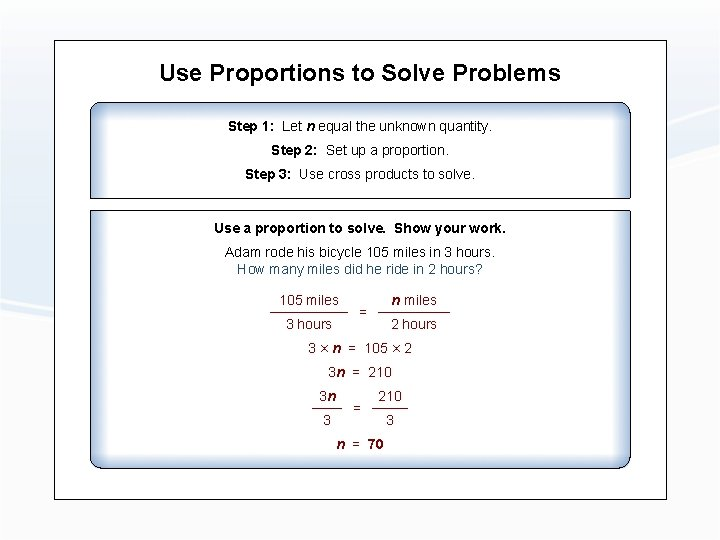# Ratios Proportions and Percents Ratios A ratio compares

• Slides: 10Ratios, Proportions, and PercentsRatios A ratio compares two quantities. A ratio can be written in three ways. Write ratios in their simplest form. The number of hearts (5) to lightning bolts (4) can be written 5: 4, 5 to 4, or 5/4. The ratio of stars (6) to lightning bolts (4) needs to be simplified. It can be written 3: 2, 3 to 2, or 3/2. Write the ratio of hearts to total objects in three ways.Ratios A ratio compares two quantities. A ratio can be written in three ways. Write ratios in their simplest form. The number of hearts (5) to lightning bolts (4) can be written 5: 4, 5 to 4, or 5/4. The ratio of stars (6) to lightning bolts (4) needs to be simplified. It can be written 3: 2, 3 to 2, or 3/2. Write the ratio of hearts to total objects in three ways. 1: 3, 1 to 3, 1/3Proportions A proportion is a statement that two ratios are equivalent. Two ratios form a proportion if: the units are the same the cross products are equal Each ratio compares hearts to lightning bolts. 5× 16 and 4× 20 are cross products. The cross products are equal.Use Proportions to Solve Problems Step 1: Let n equal the unknown quantity. Step 2: Set up a proportion. Step 3: Use cross products to solve. Problem: Jenna can buy 150 cell phone minutes for \$12. What will she pay for 225 cell phone minutes? Step 1: Let n equal the cost for 225 minutes. Step 2: = Step 3: 150 × n = 12 × 225 150 n = 2700 = n = 18 Jenna will pay \$18 for 225 minutes.Use Proportions to Solve Problems Step 1: Let n equal the unknown quantity. Step 2: Set up a proportion. Step 3: Use cross products to solve. Use a proportion to solve. Show your work. Adam rode his bicycle 105 miles in 3 hours. How many miles did he ride in 2 hours?Use Proportions to Solve Problems Step 1: Let n equal the unknown quantity. Step 2: Set up a proportion. Step 3: Use cross products to solve. Use a proportion to solve. Show your work. Adam rode his bicycle 105 miles in 3 hours. How many miles did he ride in 2 hours? 105 miles 3 hours n miles = 2 hours 3 × n = 105 × 2 3 n = 210 3 n 3 = 210 n = 70 3Percents A percent is a ratio in which the first term of the ratio is compared to 100. Example: 7% = 7 to 100, 7: 100, or 7/100 You can use a proportion to solve percent problems. What is 35% of 80? What is 12% of 125? 35% of 80 is 28. 36 is 45% of what number? 15 is what percent of 75?Percents A percent is a ratio in which the first term of the ratio is compared to 100. Example: 7% = 7 to 100, 7: 100, or 7/100 You can use a proportion to solve percent problems. What is 35% of 80? What is 12% of 125? 15 35% of 80 is 28. 36 is 45% of what number? 80 15 is what percent of 75? 20%